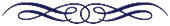# I-CHING### Iching - the oracle of changes or mutations.

It is estimated that appeared in china about 2,852 a. C.; the wise emperor Fu-Shi picked this symbolic divining technique and organized it, making the first order of the oracle. What it was a divination system transmitted orally became a book inviting to discover the great man within us. Military, kings, mystics and philosophers have used the i-ching, which when compiled into a text was consulted more often.

A hexagram is composed of six lines to be written starting from the bottom up, six lines, that each one would be solid lines or broken lines. Each hexagram is composed of two trigrams, three lines below will be a trigram and three lines above will be another trigram and this is how we will be able to identify each hexagram of the 64 which comprises the i-ching. Normally we will get a hexagram, but we can also obtain two hexagrams and would have to take one as a complementary answer, something that we have to emphasize.

We will need six runs to make a complete hexagram, the lines are becoming from the bottom up, whereby the first roll is a continuous or discontinuous line we are going to put down of all, the second straight over, the third over and so on above all until we have the sixth line. Each hexagram has an overview and then we have the descriptions of the lines that are mutants in color red, i.e. only they would only appear if we have obtained a mutant hexagram.

The side of the coin will have a score of three and each cross will have a score of two, so the lines are created based on the result of the sum of the values of heads or tails of the three coins thrown. So if the result of the sum results in an odd number, the line will be continuous and if the sum results in an even number the line will be dashed. For example, I throw the three coins, and I have two heads and one cross, would be two faces are worth three and a cross that is a two, would be 3 + 3 + 2 = 8 as the result is even then the line is discontinuous.

If there are the three coins with the same face, 3 + 3 + 3 = 9 as odd corresponds to a continuous line but also is noted as mutant, the online oracle creates that line in red. But if there are three crosses, 2 + 2 + 2 = 6 since is a pair, it corresponds a dashed red line to score that is a mutant line. Whenever the three coins are all heads or tails that line will mutate, meaning that line will become the opposite. It can be six mutants or can be none, which does not mean it is neither worse nor better, just that line want to say something exactly.

In any case, all these calculations to create the hexagrams and if it also corresponds the mutant hexagram, the oracle answers, all these processes the application of I-ching oracle is done automatically. Everything the I-ching says often happens in a very immediate time, and even sometimes warns you of things you have not even asked, the oracle basically gives good advice to help you, and reveal issues you haven't even imagine, and will surprise you.

Share in social networks